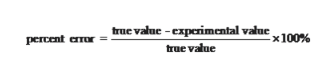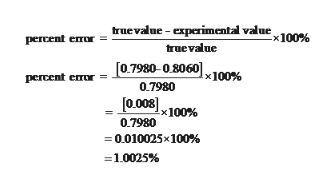Calculate the percent error for the following measurements: (a) The density of alcohol (ethanol) is found to be 0.8060 g/mL (true value = 0.7980 g/mL).  (b) The mass of gold in an earring is analyzed to be 0.8478 g (true value = 0.8644 g

Question

Calculate the percent error for the following measurements:

(a) The density of alcohol (ethanol) is found to be 0.8060 g/mL (true value = 0.7980 g/mL).

(b) The mass of gold in an earring is analyzed to be 0.8478 g (true value = 0.8644 g

Step 1

Percent error is often expressed as the absolute value of the difference between the true value and the experimental value, divided by the true value.help_outlineImage Transcriptionclosetrue value-experimental value100 percent eTor true value fullscreen
Step 2

(a)

Experimental value =0.8060 g/ml density

True value =0.7980 g/ml

Percent error of alcohol can be calculated ashelp_outlineImage Transcriptionclosetruevalue-experimental value 10026 percent emur truevalue 0.7980-0.8060100% percent error 0.7980 0.008100% 0.7980 0.010025x100 % =1.0025 % fullscreen
Step 3

(b)

Experimental value =0.8478 g/ml density

True value =0.8644 g/m...

Want to see the full answer?

See Solution

Want to see this answer and more?

Our solutions are written by experts, many with advanced degrees, and available 24/7

See Solution
Tagged in

Chemistry